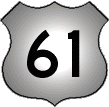# 61

This number is a prime.

Just showing those entries submitted by 'Gaydos': (Click here to show all)The reversal of 61 is a fourth power and the reversal of its fourth power is a prime. Are there any more primes with both of these properties? See answer here. [Gaydos]The sum of the lowest digit in each of the powers of two (2 + 4 + 8 + 1 + 2 + 4 + 1 + 2 + 1 + 0 + 0 + 0 + 1 + 1 + 2 + 3 + 0 ...) is 61 unless there are some large power of two that has no zero. [Gaydos]The 61st decade (601 to 610) was the first to have no semiprime year. [Gaydos]61 and its prime index (18) are both reverse fourth powers. There are no more examples of this property in the first billion primes. Are there any more at all? [Gaydos]Exactly half (61) of the first 122 emirps are distinct-digit numbers. [Gaydos]

(There are 4 curios for this number that have not yet been approved by an editor.)